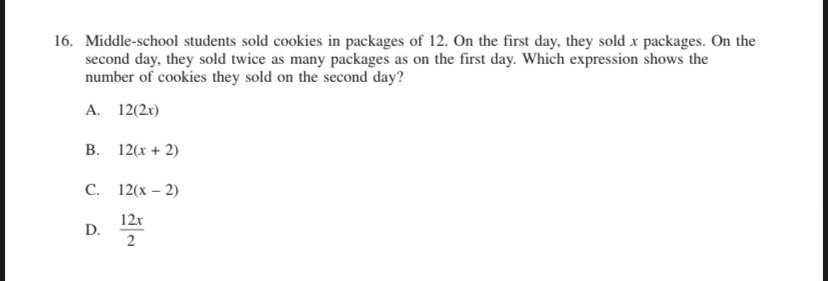### ¿Todavía tienes preguntas de matemáticas?

Pregunte a nuestros tutores expertos
Algebra
Pregunta16. Middle-school students sold cookies in packages of $$12 .$$ On the first day, they sold $$x$$ packages. On the second day, they sold twice as many packages as on the first day. Which expression shows the number of cookies they sold on the second day?

A. $$12 ( 2 x )$$

B. $$12 ( x + 2 )$$

C. $$12 ( x - 2 )$$

D. $$\frac { 12 x } { 2 }$$